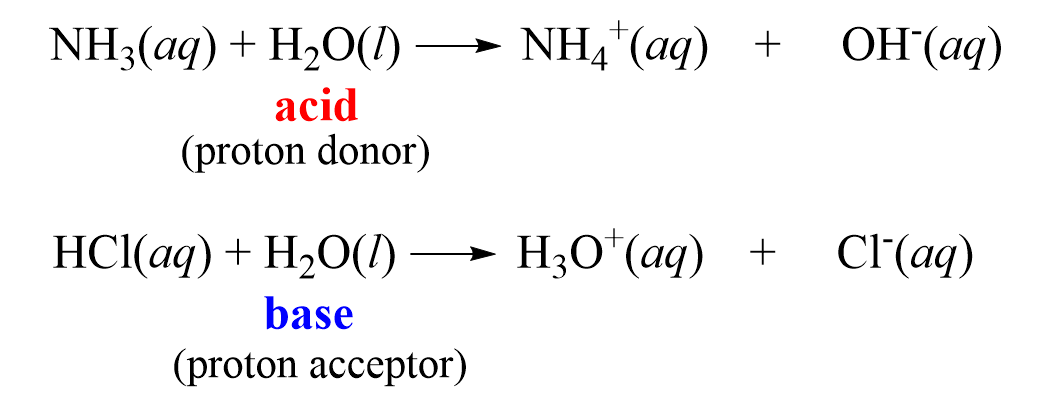## General Chemistry

We have seen several examples demonstrating that water is an amphoteric compound as it can act both as an acid and as a base. For example, it reacts with HCl as a base, and with NH3 as an acid:To generalize, we can say that in the presence of an acid, water acts as a base, while in the presence of a base, water acts as an acid.

Now, the question is can it react with itself? Can one water molecule be the acid and donate a proton to another one serving as the base?

The answer is yes, it can, and this reaction indeed occurs:

H2O(l) + H2O(l) ⇆ H3O+(aq) + OH(aq)

This is called the autoionization or dissociation of water which, in a simpler form, can be shown as:

H2O(l) ⇆ H+(aq) + OH(aq)

The equilibrium constant expression for this reaction is:

$K\, = \,{\mkern 1mu} \frac{{[{{\rm{H}}^{\rm{ + }}}][{\rm{O}}{{\rm{H}}^{\rm{ – }}}]}}{{[{{\rm{H}}_{\rm{2}}}{\rm{O}}]}}\; = \;\frac{{[{{\rm{H}}_{\rm{3}}}{{\rm{O}}^{\rm{ + }}}][{\rm{O}}{{\rm{H}}^{\rm{ – }}}]}}{{[{{\rm{H}}_{\rm{2}}}{\rm{O}}]}}$

It is determined that the concentration of water is constant (55.5 mol/l), so it is omitted from the expression, and the equilibrium constant becomes Kw which is equal to :

This equilibrium constant is called the ion product constant (dissociation constant) of water (Kw).

What is important is that the product of [H+] and [OH] is constant and equals to 10-14 at 25 oC:In pure water, the concentrations of H+ and OH ions are equal, so by taking the square root of Kw, the concentration of H+ and OH ions in water are found to be:

${\rm{[}}{{\rm{H}}^{\rm{ + }}}{\rm{]}}\; = \;{\rm{[O}}{{\rm{H}}^{\rm{ – }}}{\rm{]}}\;{\rm{ = }}\;\sqrt {{K_{\rm{w}}}} \; = \;1.0\, \times \,{10^{ – 7}}\,M$

When [H+] = [OH], we have a neutral solution, when [H+] > [OH] the solution becomes acidic, and if [H+] < [OH], then it is a basic solution.

We can change the concentrations of H+ and OH but because they are correlated and their product is constant, we cannot do it independently. For example, if we increase [H+], the [OH] will decrease and vice versa.

Given the [H+] or [OH-], we can calculate the other one.

For example, if [H+] = 10-3, we can determine the [OH] using the expression for Kw:

Kw = [H+][OH], therefore,

${\rm{[O}}{{\rm{H}}^{\rm{ – }}}{\rm{]}}\;{\rm{ = }}\;\frac{{\sqrt {{K_{\rm{w}}}} \;}}{{{\rm{[}}{{\rm{H}}^{\rm{ + }}}{\rm{]}}\;}}\; = \;\frac{{{{10}^{ – 14}}\;}}{{{{10}^{ – 3}}\;}}\, = 1.0\, \times \,{10^{ – 11}}\,M$

So, this is an acidic solution because [H+] > [OH].

In the next article, we will talk about the pH scale as an indicator of the acidity or basicity of the solution.

Check Also